Important Coordinate Geometry Questions

Chapter 7 Class 10 Coordinate Geometry
Serial order wise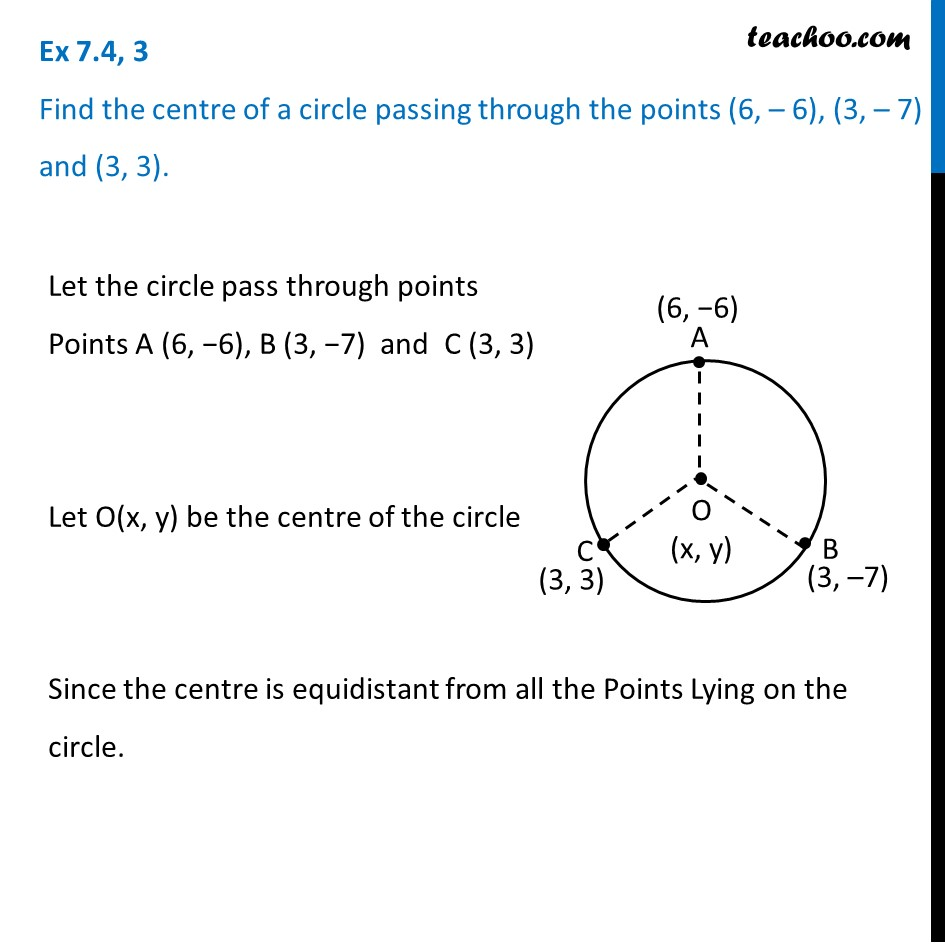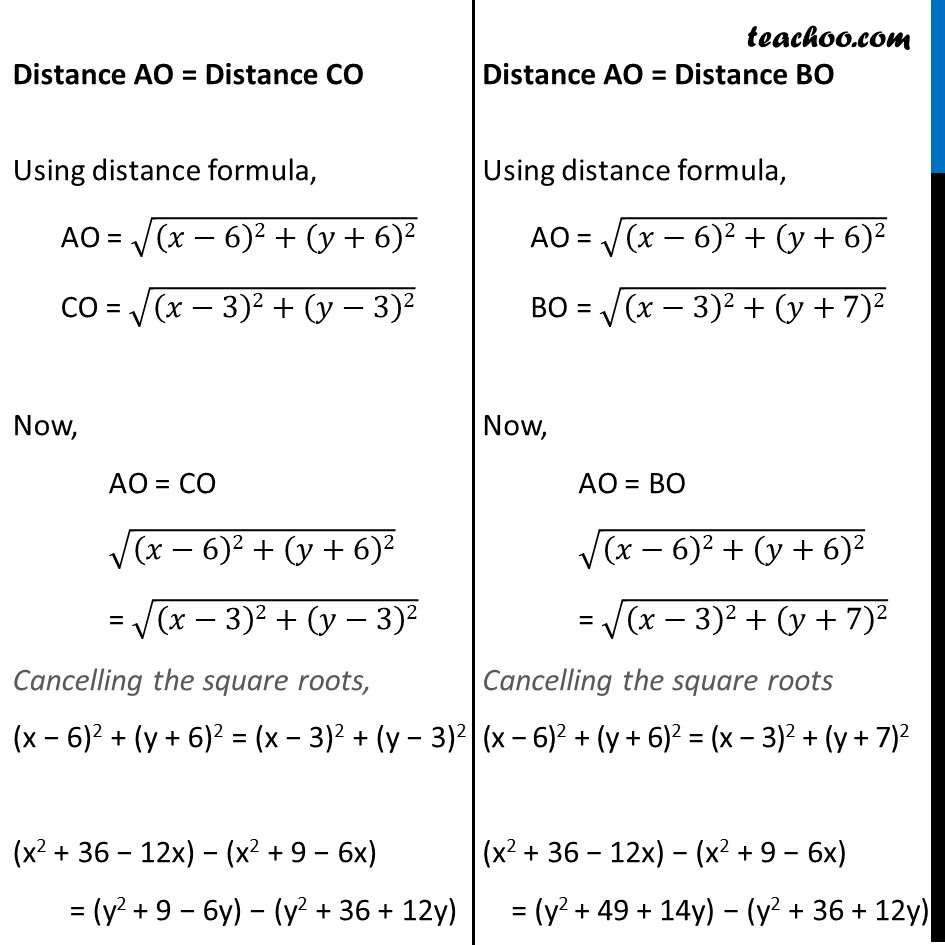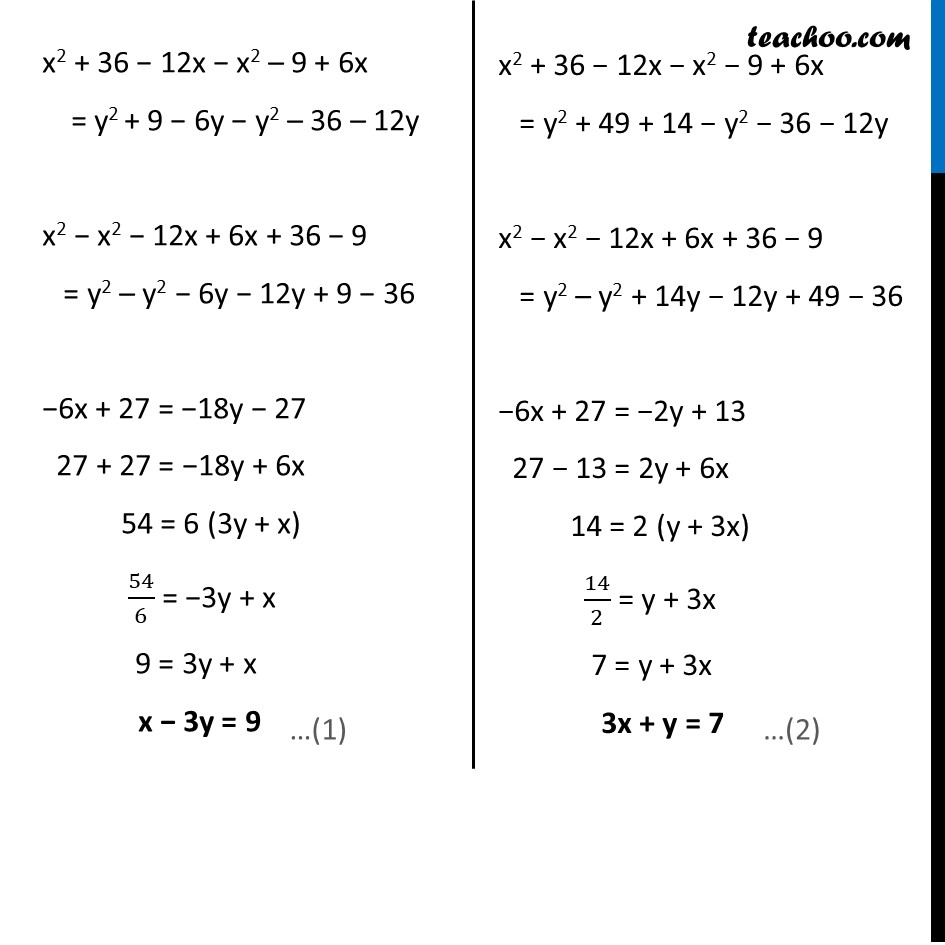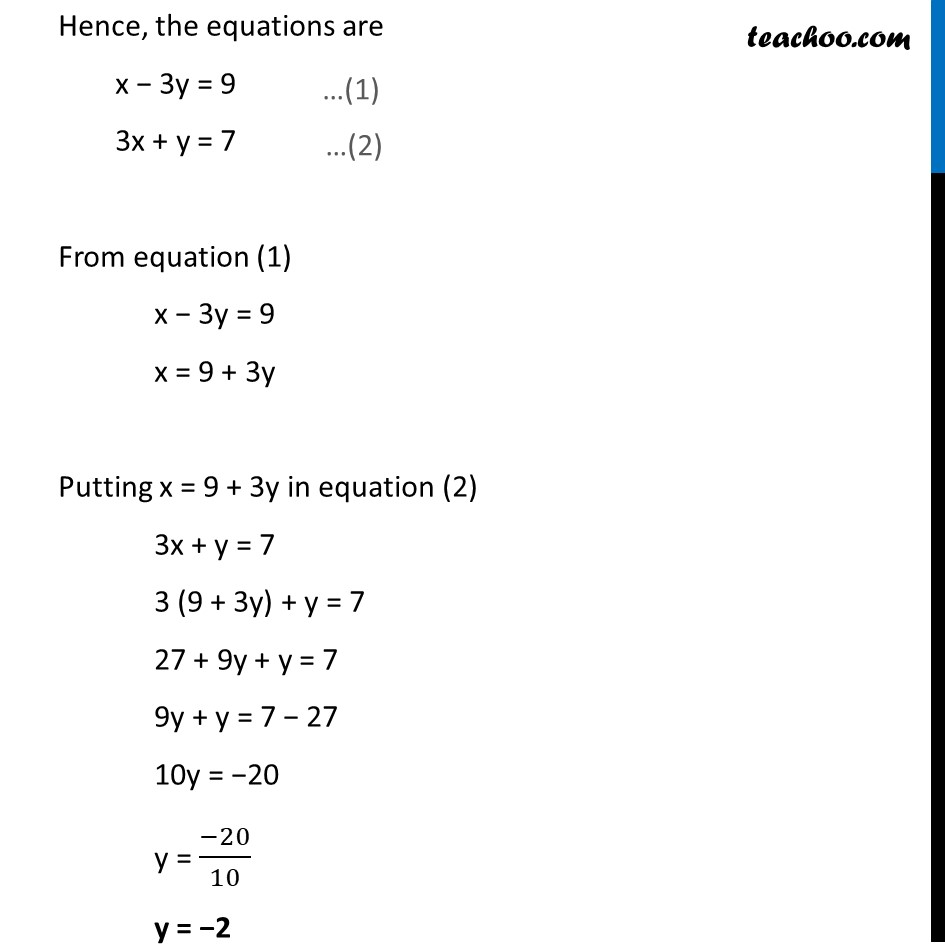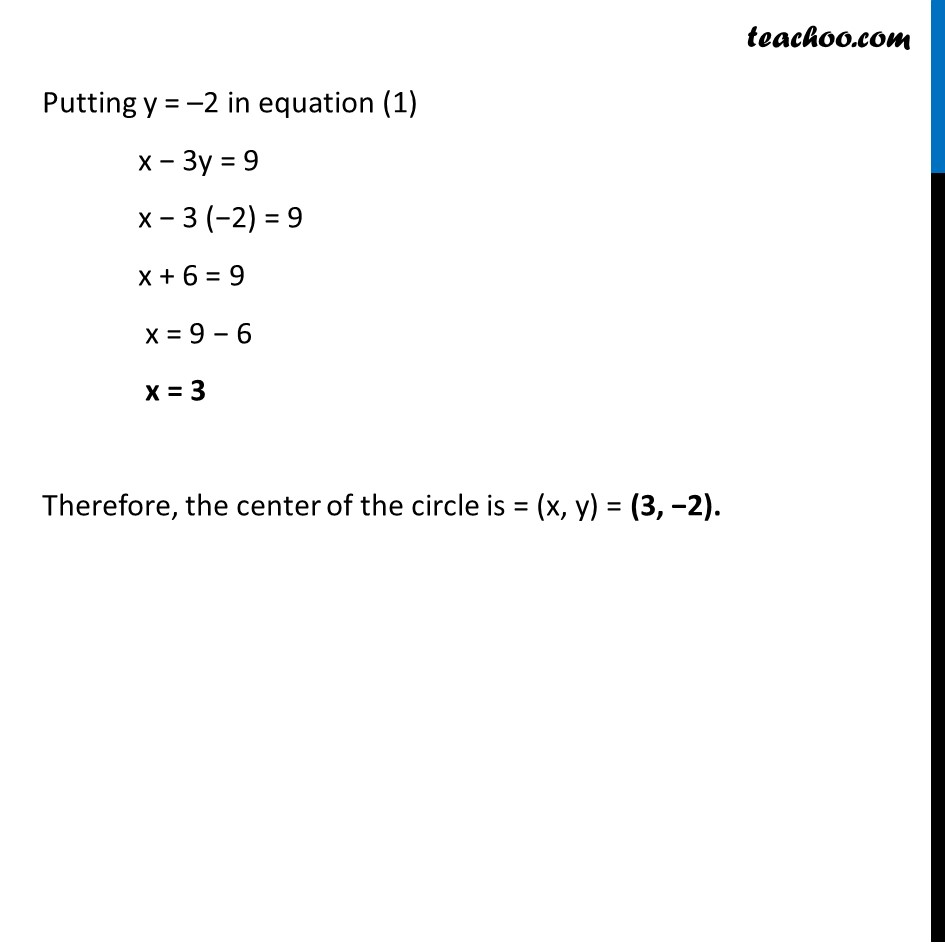Learn in your speed, with individual attention - Teachoo Maths 1-on-1 Class

### Transcript

Question 3 Find the centre of a circle passing through the points (6, – 6), (3, – 7) and (3, 3). Let the circle pass through points Points A (6, −6), B (3, −7) and C (3, 3) Let O(x, y) be the centre of the circle Since the centre is equidistant from all the Points Lying on the circle. Distance AO = Distance CO Using distance formula, AO = √((𝑥−6)2+(𝑦+6)2) CO = √((𝑥−3)2+(𝑦−3)2) Now, AO = CO √((𝑥−6)2+(𝑦+6)2) = √((𝑥−3)2+(𝑦−3)2) Cancelling the square roots, (x − 6)2 + (y + 6)2 = (x − 3)2 + (y − 3)2 (x2 + 36 − 12x) − (x2 + 9 − 6x) = (y2 + 9 − 6y) − (y2 + 36 + 12y) Distance AO = Distance BO Using distance formula, AO = √((𝑥−6)2+(𝑦+6)2) BO = √((𝑥−3)2+(𝑦+7)2) Now, AO = BO √((𝑥−6)2+(𝑦+6)2) = √((𝑥−3)2+(𝑦+7)2) Cancelling the square roots (x − 6)2 + (y + 6)2 = (x − 3)2 + (y + 7)2 (x2 + 36 − 12x) − (x2 + 9 − 6x) = (y2 + 49 + 14y) − (y2 + 36 + 12y) x2 + 36 − 12x − x2 – 9 + 6x = y2 + 9 − 6y − y2 – 36 – 12y x2 − x2 − 12x + 6x + 36 − 9 = y2 – y2 − 6y − 12y + 9 − 36 −6x + 27 = −18y − 27 27 + 27 = −18y + 6x 54 = 6 (3y + x) 54/6 = −3y + x 9 = 3y + x x − 3y = 9 x2 + 36 − 12x − x2 − 9 + 6x = y2 + 49 + 14 − y2 − 36 − 12y x2 − x2 − 12x + 6x + 36 − 9 = y2 – y2 + 14y − 12y + 49 − 36 −6x + 27 = −2y + 13 27 − 13 = 2y + 6x 14 = 2 (y + 3x) 14/2 = y + 3x 7 = y + 3x 3x + y = 7 Hence, the equations are x − 3y = 9 3x + y = 7 From equation (1) x − 3y = 9 x = 9 + 3y Putting x = 9 + 3y in equation (2) 3x + y = 7 3 (9 + 3y) + y = 7 27 + 9y + y = 7 9y + y = 7 − 27 10y = −20 y = (−20)/10 y = −2 Putting y = –2 in equation (1) x − 3y = 9 x − 3 (−2) = 9 x + 6 = 9 x = 9 − 6 x = 3 Therefore, the center of the circle is = (x, y) = (3, −2).